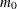Displayed Output

The output produced by PROC MULTTEST is divided into several tables. If the DATA= data set is specified, then the following tables are displayed:

• The Model Information table provides a list of the options and settings used for that particular invocation of the procedure. This table is not displayed if the INPVALUES= data set is specified. Included in this list are the following items:

• statistical tests

• support of the exact permutation distribution for the CA and Peto tests

• continuity corrections used for the CA test

• test tails

• p-value adjustments and specified suboptions

• centering of continuous variables

• number of samples and seed

• The Contrast Coefficients table lists the coefficients used in constructing the statistical tests. These coefficients are either specified in CONTRAST statements or generated by default. The coefficients apply to the levels of the CLASS statement variable. If a MEAN or FT test is specified in the TEST statement, the centered coefficients are displayed. Patterns of missing values in your data set might affect the coefficients used in your analysis; the displayed contrasts take missing value patterns into account. See the section Missing Values for more information.

• The Variable Tabulations tables provide summary statistics for each variable listed in the TEST statement. Included for discrete variables are the count, sample size, and percentage of occurrences. For continuous variables, the mean, sample standard deviation, and sample size are displayed. All of the previously mentioned statistics are computed for distinct combinations of the CLASS and STRATA statement variables.

If the INPVALUES= data set is specified, then the following tables are displayed:

• whether the finite-sample version of the PFDR is used (FINITE)

• the number of tuning parameters to check (NLAMBDA=), the maximum tuning parameter (MAXLAMBDA=), or the specified tuning parameter (LAMBDA=)

• the degrees of freedom of the spline (DF=) and the smoothing parameter

• the number of bootstrap resamples (NBOOT=) and the seed (SEED=)

• If the bootstrap or spline method for estimating the number of true null hypothesesis used and the PLOTS= option is specified, the Lambda Values table displays theestimates as a function of the tuning parameter. If the bootstrap method is used, the table also displays the mean-squared errors, the minimum of which is used to select a specific. This table contains the values used in the Lambda Functions plot.

• The Estimated Number of True Null Hypotheses table displays the p-value adjustment, the method used to estimate the number of true nulls, and an estimate of the number and proportion of true null hypotheses in the data set.

The following table is displayed unless the NOPVALUE option is specified:

• The p-Values table is a collection of the raw and adjusted p-values from the run of PROC MULTTEST. The p-values are identified by variable and test.